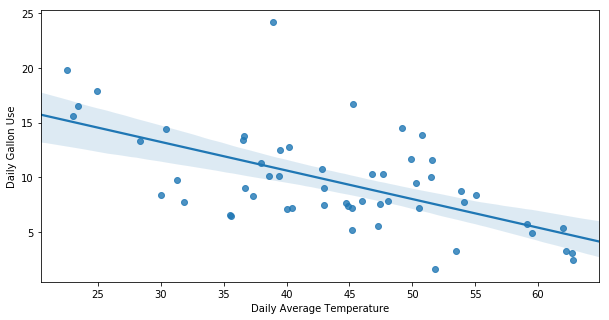I recently moved to a house that uses heating oil. The tank has a wireless sensor that transmits the number of gallons remaining in the tank via the provider's website. I was interested in estimating how many gallons of oil we use based on the temperature outside. This requires two steps: record the daily value of gallons remaining in the tank and regress it against the temperature outside.

1. Scraping your data: The code on this Github page shows how to use the Python selenium package to log into your account, access the appropriate page and extract the field called gallons. A daily cron job runs the code, see this for creating a job on a Mac.
2. Linear Regression: External temperature is pulled from this site as a csv. A linear relationship is estimated using the daily gallons and temperature recorded. In other words, daily gallons used = daily gallons used at 0 deg F + temperature x daily gallons used per degree. This is estimated using sklearn and the constants are: daily gallons used at 0F is ~20 gallons and gallons used per degree = -0.2 gallons per degree. In other words, if it is 30F outside, we expect to use about 15 gallons.
3. K-factor and degree days: You can also calculate a home's k-factor, a measure of efficiency. This number is most useful when comparing numbers to other homes, like your neighborhood. Roughly, you take the sum of degree days and divide it by the total gallons you use. Degree days just 65 - average temperature fo that day. So, using 15 gallons on a day that is 30 degrees is a k-factor of about 2, which is extremely inefficient. While it depends on square footage, new houses can be on the order of 6.